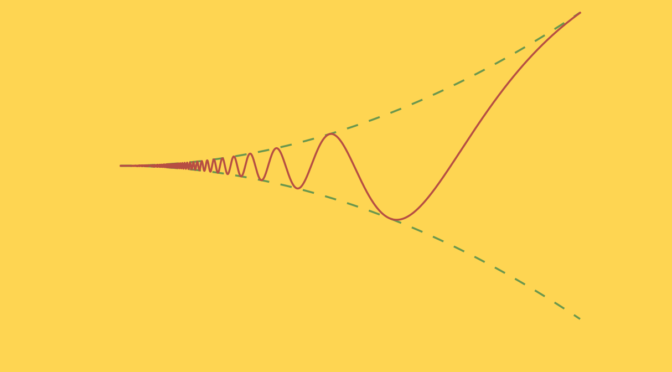# A differentiable real function with unbounded derivative around zero

Consider the real function defined on $$\mathbb R$$$f(x)=\begin{cases} 0 &\text{for } x = 0\\ x^2 \sin \frac{1}{x^2} &\text{for } x \neq 0 \end{cases}$

$$f$$ is continuous and differentiable on $$\mathbb R\setminus \{0\}$$. For $$x \in \mathbb R$$ we have $$\vert f(x) \vert \le x^2$$, which implies that $$f$$ is continuous at $$0$$. Also $\left\vert \frac{f(x)-f(0)}{x} \right\vert = \left\vert x \sin \frac{1}{x^2} \right\vert \le \vert x \vert$ proving that $$f$$ is differentiable at zero with $$f^\prime(0) = 0$$. The derivative of $$f$$ for $$x \neq 0$$ is $f^\prime(x) = \underbrace{2x \sin \frac{1}{x^2}}_{=g(x)}-\underbrace{\frac{2}{x} \cos \frac{1}{x^2}}_{=h(x)}$ On the interval $$(-1,1)$$, $$g(x)$$ is bounded by $$2$$. However, for $$a_k=\frac{1}{\sqrt{k \pi}}$$ with $$k \in \mathbb N$$ we have $$h(a_k)=2 \sqrt{k \pi} (-1)^k$$ which is unbounded while $$\lim\limits_{k \to \infty} a_k = 0$$. Therefore $$f^\prime$$ is unbounded in all neighborhood of the origin.